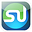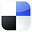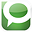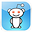# Regression AnalysisDone in Excel2 Most Important Steps

Running a Regression in Excel is fairly easy. So is running one incorrectly. There are two crucial steps that should always be performed on the data before any Regression should be run. Fortunately these two steps are very quick and easy to do in Excel. They are:

1) Graph the Data
2) Run Correlation Analysis On All Variables

Here is a video of this article showing how to perform all four steps to Regression in Excel, including the above two crucial steps at the beginning:

Step-By-Step Video Showing How To Do All 4 Steps of Regression in Excel, Including the 2 Crucial Initial Steps That No One Does, But Should

Why You Need To Run The 2 Crucial Steps Before Doing Regression

Here’s why you need to run the two crucial steps prior to regressing any data in Excel:

Crucial Step 1) Graphing the Data

Whether or not you are using Excel to run a Regression, you should always graph the data before doing anything else. Eyeballing the data will allow you to quickly determine whether there is any relationship between the independent (input) variables and the dependent (output) variable. You also want to evaluate whether the graph generally appears to be linear or possibly quadratic. Excel’s Regression Tool works well only for reasonably linear data. Eyeballing the data upfront will tell you very quickly whether Excel’s Linear Regression is the right tool for the job.

Graphing The Data To Check If It Is Linear
Click On Image To See Enlarged View

The input and output variables will be graphed together. The y-axis of the chart will provide the scale for plotting of those values. The x-axis will provide a measure of whatever continuum was used, e.g. time, to collect the values of all of the variables. Excel’s charting function is the way to go here. The above linked video shows exactly how to chart all the data in Excel.

Crucial Step 2) Running Correlation Analysis on All Variables Simultaneously

There are two good reasons for doing this. First, we want to remove any input variables which are clearly not good predictors of the output variable. Second, we want to make sure that none of the input variables have a high correlation with (are good predictors of) other input variables.

Running Correlation Analysis on the Data To Prevent Collinearity and also To Remove Input Variables That Have Low Correlation With the Output Variable
Click On Image To See Enlarged View

Correlation of multiple variables is easily done in Excel using the Correlation Data Analysis tool. The linked video shows exactly how to do that.

Remove Input Variables That Have Low Correlation With Output Variable

After you have run Correlation Analysis on the data, you will want to remove any input variables that have a low correlation with the output variable. A Correlation Coefficient of with an absolute value of less than 0.4 (between -0.4 and +0.4) between the output variable and an input variable indicates that the input variable is not a good predictor of the output. That input variable should be removed from the Regression Analysis. The attached video provides an example of this.

Data Columns Before Removing Input Variable With Low Correlation To Output

Click On Image To See Enlarged View

Data Columns After Removing Input Variables With Low Correlation To Output

Click On Image To See Enlarged View

Remove Inputs Variables Highly Correlated With Other Input Variables

After looking at the Correlation Coefficients between the input and output variables, look at the Correlation Coefficients between the input variables themselves. You do not want to use pairs of input variables that are good predictors of each other in a Regression. This will cause a Regression error known as Collinearity or Multicollinearity. One variable from any pair of highly-correlated input variables should be removed prior to running the Regression Analysis. Variables can be considered highly-Correlated if the absolute value of their Correlation Coefficient is greater the 0.7 (greater than +0.7 or less than -0.7).

Adding New Input Variables To The Regression Analysis

Here are a few hints about adding new input variables to a Regression Analysis. First, build up a Regression by starting with a small number of input variables and add any new ones one at a time. Second, good new input variables noticeably increase Adjusted R Square and also lower Standard Error without significantly changing the existing Regression Coefficients.

When you are satisfied with the output of the data graph and the Correlation Analysis, go ahead and run the Regression with Excel. An example of how to do this is shown in the above video.

The Excel Regression Dialogue Box
Click On Image To See Enlarged View

The final step of Excel Regression is Analysis of the Excel output. Here is a link to another video which shows you how to quickly read the most important parts of the Excel Regression output: http://bit.ly/Quickly-Understanding-Excel-Regression-Output

Excel Regression Output With Color Coding Added
Click On Image To See Enlarged View

Conclusion - Plotting the Data and Running Correlation Can Be BIG Time Savers

Plotting the data and running Correlation Analysis prior to running a Regression can save you lots of time that you might otherwise have to spend making adjustments to your Regression after running it.

If You Like This, Then Share It...Excel Master Series Blog Directory

Statistical Topics and Articles In Each Topic

• Histograms in Excel
• Bar Chart in Excel
• Combinations & Permutations in Excel
• Normal Distribution in Excel
• t-Distribution in Excel
• Binomial Distribution in Excel
• z-Tests in Excel
• t-Tests in Excel
• Hypothesis Tests of Proportion in Excel
• Chi-Square Independence Tests in Excel
• Chi-Square Goodness-Of-Fit Tests in Excel
• F Tests in Excel
• Correlation in Excel
• Pearson Correlation in Excel
• Spearman Correlation in Excel
• Confidence Intervals in Excel
• Simple Linear Regression in Excel
• Multiple Linear Regression in Excel
• Logistic Regression in Excel
• Single-Factor ANOVA in Excel
• Two-Factor ANOVA With Replication in Excel
• Two-Factor ANOVA Without Replication in Excel
• Creating Interactive Graphs of Statistical Distributions in Excel
• Solving Problems With Other Distributions in Excel
• Optimization With Excel Solver
• Chi-Square Population Variance Test in Excel
• Analyzing Data With Pivot Tables
• SEO Functions in Excel
• Time Series Analysis in Excel

# Regression AnalysisDone in ExcelHow To Read the Output

There is a lot more to the Excel Regression output than just the regression equation. If you know how to quickly read the output of a Regression done in, you’ll know right away the most important points of a regression: if the overall regression was a good, whether this output could have occurred by chance, whether or not all of the independent input variables were good predictors, and whether residuals show a pattern (which means there’s a problem).

Excel Regression Output With Color-Coding Added

Click On Image To See Enlarged View

This video will illustrate exactly how to quickly and easily understand the output of Regression performed in Excel:

Step-By-Step Video About How To Quickly Read and Understand the Output of Excel Regression

The 4 Most Important Parts of Regression Output
1) Overall Regression Equation’s Accuracy
(R Square and Adjusted R Square)

2) Probability That This Output Was Not By Chance
(ANOVA – Significance of F)

3) Individual Regression Coefficient and Y-Intercept Accuracy

4) Visual Analysis of Residuals

Some parts of the Excel Regression output are much more important than others. The goal here is for you to be able to glance at the Excel Regression output and immediately understand it, so we will focus our attention only on the four most important parts of the Excel regression output.

1) Overall Regression’s Accuracy

Click On Image To See Enlarged View

R Square– This is the most important number of the output. R Square tells how well the regression line approximates the real data. This number tells you how much of the output variable’s variance is explained by the input variables’ variance. Ideally we would like to see this at least 0.6 (60%) or 0.7 (70%).

Adjusted R Square – This is quoted most often when explaining the accuracy of the regression equation. Adjusted R Square is more conservative the R Square because it is always less than R Square. Another reason that Adjusted R Square is quoted more often is that when new input variables are added to the Regression analysis, Adjusted R Square increases only when the new input variable makes the Regression equation more accurate (improves the Regression equations’s ability to predict the output). R Square always goes up when a new variable is added, whether or not the new input variable improves the Regression equation’s accuracy.

2) Probability That This Output Was Not By Chance

Click On Image To See Enlarged View

Significance of F
– This indicates the probability that the Regression output could have been obtained by chance. A small Significance of F confirms the validity of the Regression output. For example, if Significance of F = 0.030, there is only a 3% chance that the Regression output was merely a chance occurrence.

3) Individual Regression Coefficient Accuracy

Click On Image To See Enlarged View

P-value of each coefficient and the Y-intercept – The P-Values of each of these provide the likelihood that they are real results and did not occur by chance. The lower the P-Value, the higher the likelihood that that coefficient or Y-Intercept is valid. For example, a P-Value of 0.016 for a regression coefficient indicates that there is only a 1.6% chance that the result occurred only as a result of chance.

4) Visual Analysis of Residuals

Charting the Residuals
Click On Image To See Enlarged View

The Residual Chart
Click On Image To See Enlarged View

The residuals are the difference between the Regression’s predicted value and the actual value of the output variable. You can quickly plot the Residuals on a scatterplot chart. Look for patterns in the scatterplot. The more random (without patterns) and centered around zero the residuals appear to be, the more likely it is that the Regression equation is valid.

There are many other pieces of information in the Excel regression output but the above four items will give a quick read on the validity of your Regression.

If anyone has any comments or observations related to this article, feel free to submit them because your input and opinions are highly valued.

If You Like This, Then Share It...Excel Master Series Blog Directory

Statistical Topics and Articles In Each Topic

• Histograms in Excel
• Bar Chart in Excel
• Combinations & Permutations in Excel
• Normal Distribution in Excel
• t-Distribution in Excel
• Binomial Distribution in Excel
• z-Tests in Excel
• t-Tests in Excel
• Hypothesis Tests of Proportion in Excel
• Chi-Square Independence Tests in Excel
• Chi-Square Goodness-Of-Fit Tests in Excel
• F Tests in Excel
• Correlation in Excel
• Pearson Correlation in Excel
• Spearman Correlation in Excel
• Confidence Intervals in Excel
• Simple Linear Regression in Excel
• Multiple Linear Regression in Excel
• Logistic Regression in Excel
• Single-Factor ANOVA in Excel
• Two-Factor ANOVA With Replication in Excel
• Two-Factor ANOVA Without Replication in Excel
• Creating Interactive Graphs of Statistical Distributions in Excel
• Solving Problems With Other Distributions in Excel
• Optimization With Excel Solver
• Chi-Square Population Variance Test in Excel
• Analyzing Data With Pivot Tables
• SEO Functions in Excel
• Time Series Analysis in Excel